# "Spirals of Harris" thought inspired by the beauty that the golden ratio produces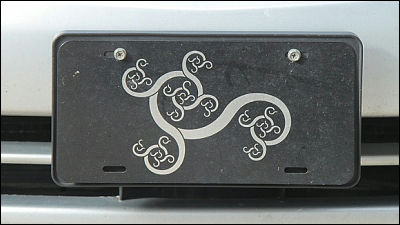Mathematics dealing with numbers is often regarded as mechanical and inorganic, but in reality it may have elements that are surprisingly aesthetic, and from a long time agoMathematical beautyThere are,Music and MathematicsIt was also thought that it was related. Edmund Harris Assistant Professor at the University of ArkansasGolden ratioHe got a hint on the figure that he draws and succeeded in drawing a new figure that did not exist so far.

The golden ratio has spawned a beautiful new curve: the Harriss spiral | Alex Bellos | Science | The Guardian

Mr. Edmund Harris who devised a new figure is an assistant professor at the Arts Sciences Department of Arts and Sciences, University of Arkansas. It is also a person who is producing a work of art created by figures.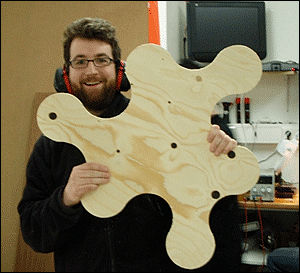Here is the figure that Mr. Harris invented newly. Part and whole resemblefractalThis figure is based on the Golden RatioGolden spiralIt has been arranged as a base.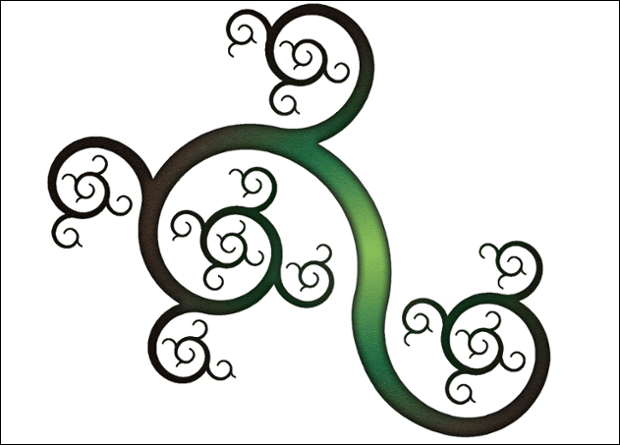◆ What is a golden spiral?
It became the basis of figures that the ratio of the length to the width is the golden ratio of 1.618Golden Rectangleis. The golden rectangle has the property that when drawing a square (white part) in it, the remaining part (blue) turns into a golden rectangle again.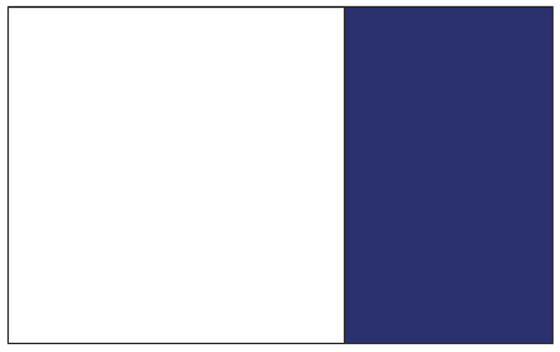If you divide the newly created golden rectangle in the same way as above, a new golden rectangle will appear again. In this way, it is possible to divide the golden rectangle into infinite small pieces.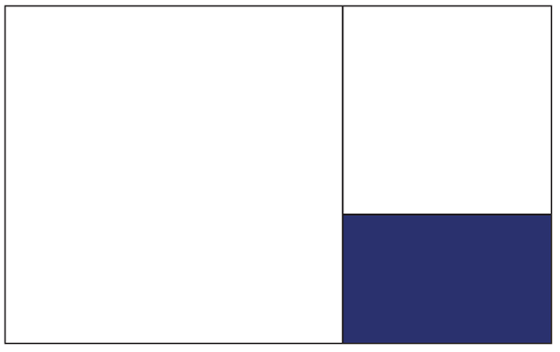Next, in each square, twoDiagonalGo throughQuadrant(Shibusen) is drawn, and as a circular arc is connected, a spiral helix (helix) gradually becoming smaller (larger) is drawn. The spiral drawn in this way is called "Golden spiralIt is also a famous figure often used in explaining the golden rule. (However, the correct golden spiral is an equiangular spiral whose curvature changes smoothly, and the one shown in this figure is not strictly a golden spiral, but it is omitted here)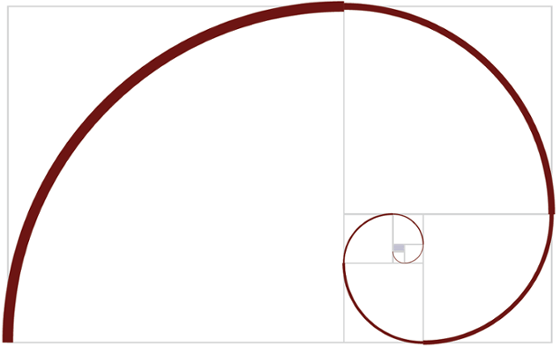◆ Arrange based on golden spirals
It is said that the golden ratio has been used since the period of ancient Greece, and it is said that the influence can be found also in the famous Parthenon temple and the pyramid. Mr. Harris brings a new attempt to the golden rectangle and the golden spiral based on such a golden ratio.

Mr. Harris talks about that attempt, "I drew a rectangle instead of drawing a square." The figure below shows how to divide, but here Mr. Harris first drew two similar rectangles (blue and orange), and as a result we found a division method where the square (white) remains. The rectangle drawn here differs from the golden ratio (1.618) by "1.325It has an aspect ratio of "It is an aspect ratio.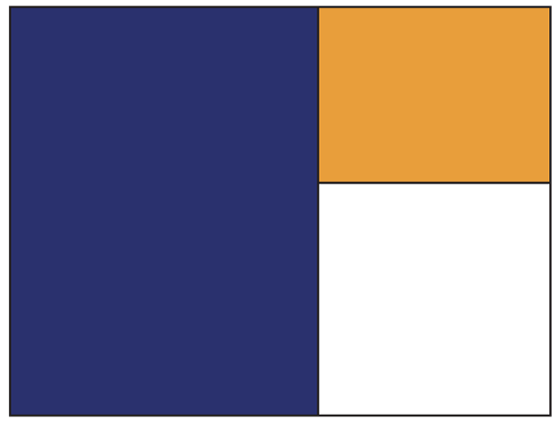I will divide the rectangle drawn in this way with the same ratio.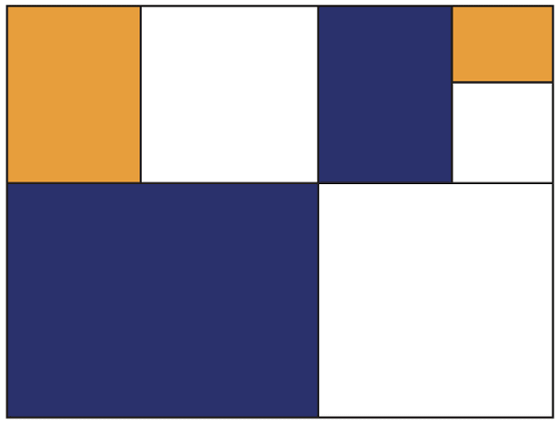Further break up and down. At this point, the figure is showing the aspect of fractal.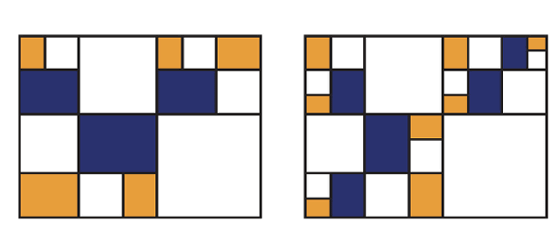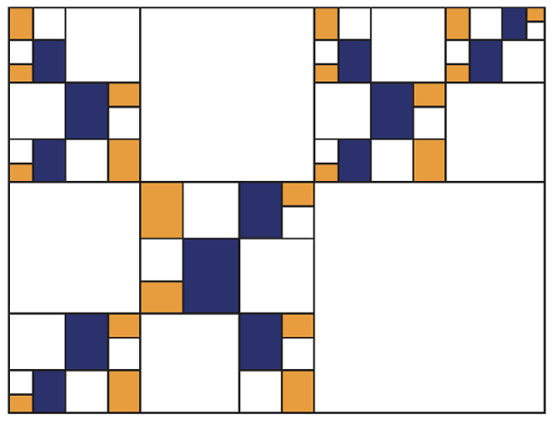Here Mr. Harris is not a diagonal with a white squareQuadrant passing through two adjacent cornersI will bring in a new attempt to draw. Although it is drawn like a golden spiral when it is seen at a glance, it is important point that a white square which does not draw a circular arc is left in the figure yet.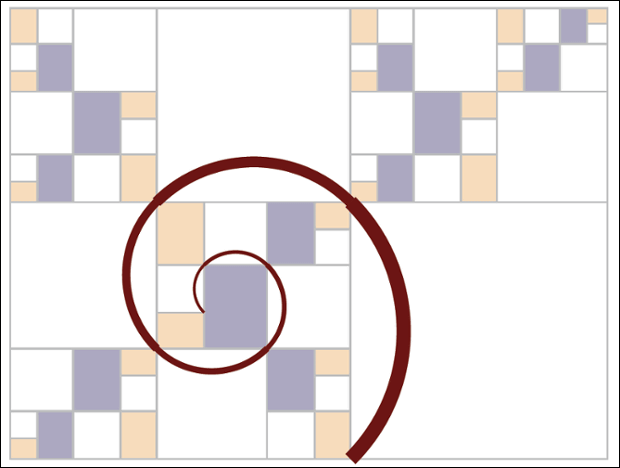When drawing circular arcs in all squares in the same way, several small spirals that "branched" one after another appeared. A golden spiral was drawn with one equiangular circle spreading from the center, Mr. Harris draws a fractal figure in which circular arcs with the same shape appear one after another.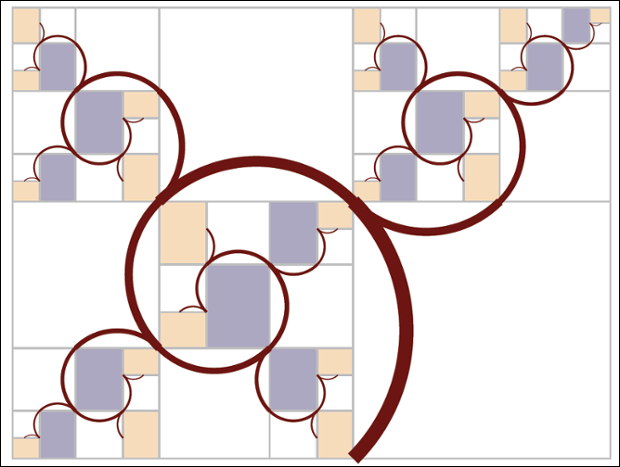Finally, when we erase the largest circular arc, a figure composed of a spiral has been completed. This is the figure to call "Harris's spiral" created by Harris.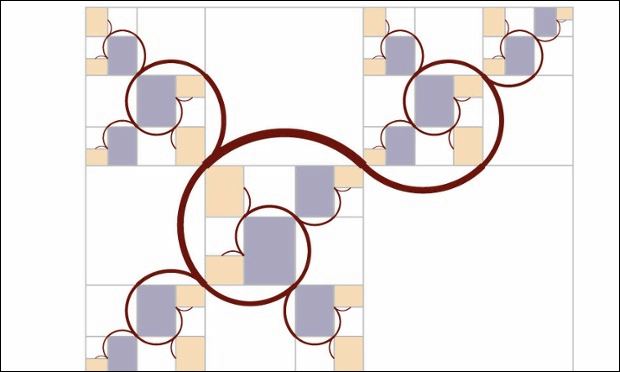When I saw this figure, Mr. Harris said that he was delighted with its beauty. Mr. Harris' first aim is to make Islamic art andKlimtIt seems that it was to draw a branching spiral as seen in the work of Mr. Nakajima, but he seems to be surprised that it was born through a mathematical process. Mr. Harris said that this figure was born, "Making things that none have seen is not that hard, but it is truly difficult, as it is mathematical but new things that no one has seen It is to make up. "

In fact, Mr. Harris seemed worried that there was an example drawn by this method, "The difficult point of mathematical discovery and mathematical art is that even if the method is totally new, that Is not to guarantee that "never tried anyone before", he said.

The proportion of the rectangle that gave rise to "Harris's spiral" was "1.325" as mentioned above, but this is the mathematical pointNumber of plasticsIt turned out that it was never drawn figures using this number so far. It turned out that the "spiral of Harris" was of authentic Harris' idea. Harris' spirals are drawn on T - shirts ......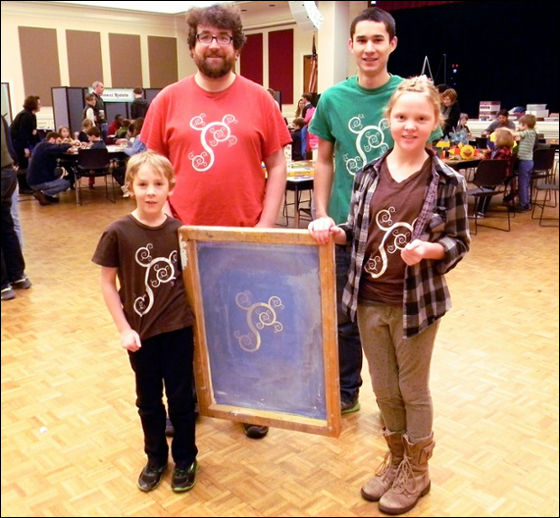It has been drawn on cards and Harris's car license plate holder.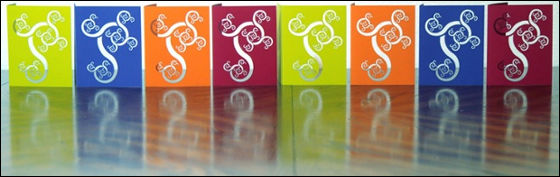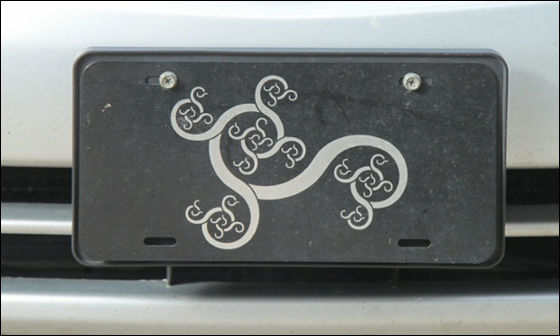◆ Geometry drawn by other ratio
Mr. Harris is further addressing that Harris is bringing it into the spreading geometry method called "Proportion Systems (ratio system)". It seems to be to obtain the proportion of figures that similar figures continue to appear even if one figure is repeatedly divided, but the principle of the figure is "to square and to similar rectangleonlyRectangle to be divided ". It is known that geometries satisfying this principle are limited to only three geometries with the following ratio. A figure with a ratio of 1.41421 (= √ 2) on the upper left has the same ratio as that of "A row" paper such as "A4 paper". The figure on the upper right is a figure with the so-called golden rule, the figure on the bottom is a simple one with two squares side by side.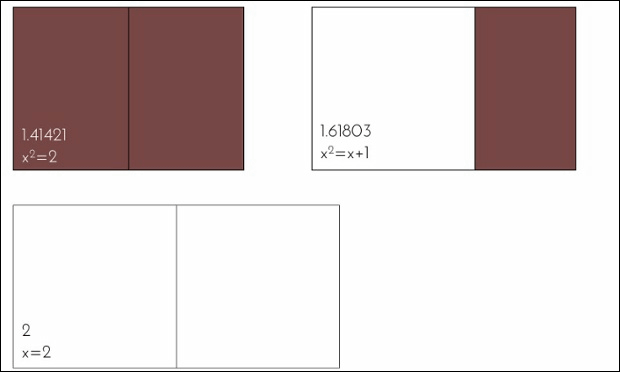Furthermore, when dividing it into three using the same principle, 16 shapes are possible. Among them, there are six combinations of squares that can be divided into one and two similar rectangles as follows.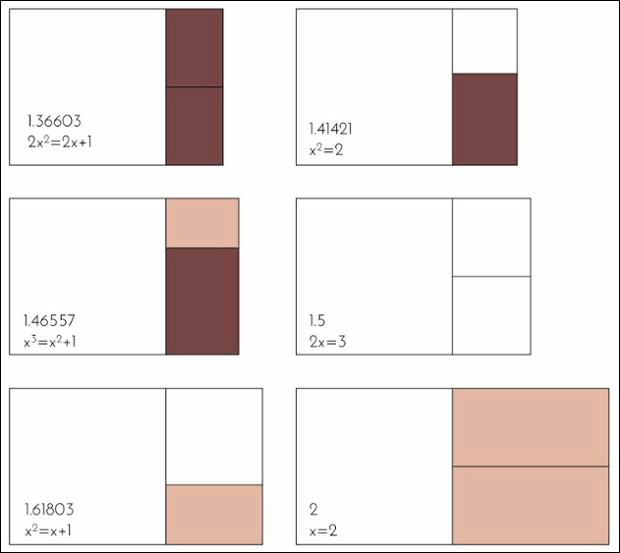Also, it has similar rectangle, and combinations of rectangle and square are aligned on the right side in the following six ways. The combination of the lower left is a combination that produced the above "Harris' spiral".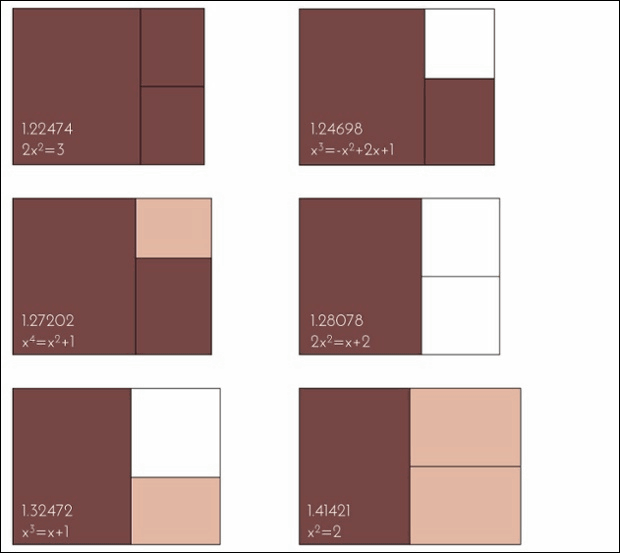And the three figures are aligned horizontally in the following four ways.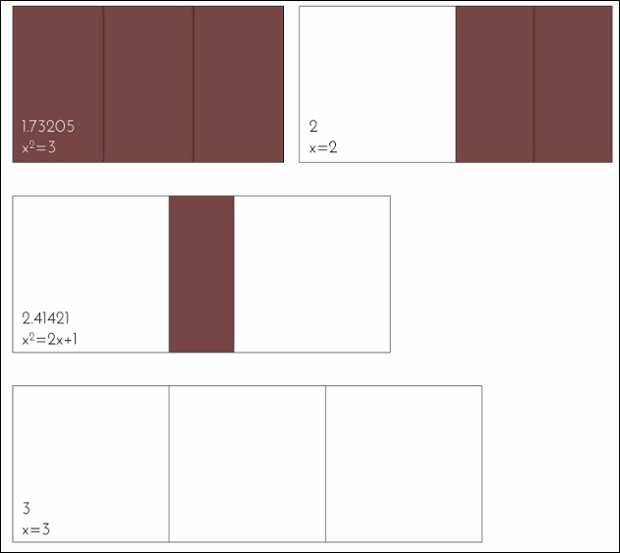If you are a bright person in mathematics, the numbers shown in the illustration aboveAlgebraic numberYou may notice that. Mr. Harris believes that this geometric approach further considers that understanding will advance.

In the spirals drawn in this way, besides Harris' spiral, the following things are born. It seems to be difficult to produce many patterns, but it seems to be said that it is an example that mathematics feels that it has the power of magical art.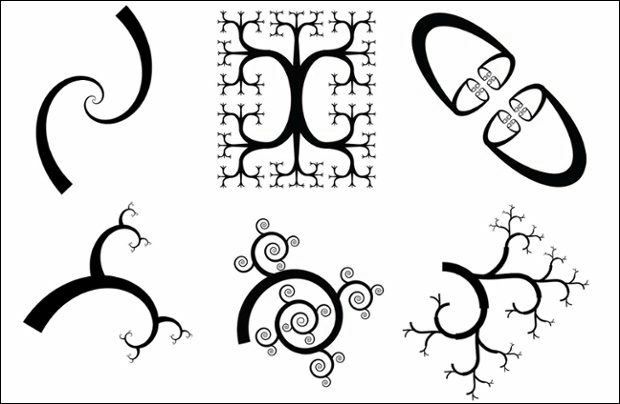Mr. Harris's page contains numerous figures drawn on the basis of mathematical theory and it seems to be interesting for those who are interested.

Edmund Harriss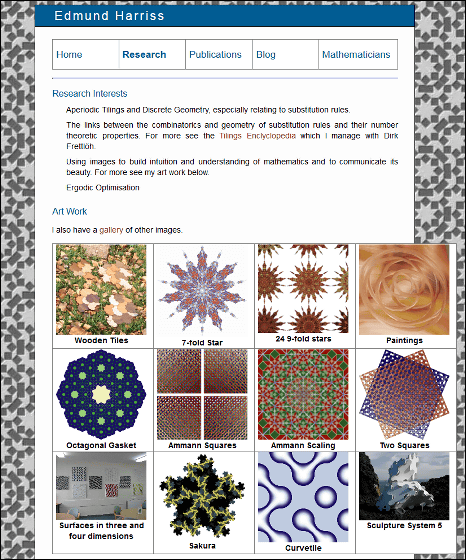in Science,   Design,   Art, Posted by darkhorse_log